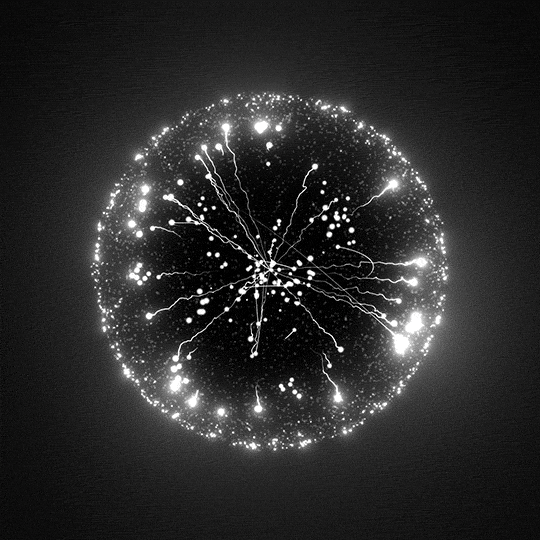# Particle Swarm OptimizationIn computational science, particle swarm optimization (PSO) is a computational method that optimizes a problem by iteratively trying to improve a candidate solution with regard to a given measure of quality. It solves a problem by having a population of candidate solutions, here dubbed particles, and moving these particles around in the search-space according to simple mathematical formula over the particle's position and velocity. Each particle's movement is influenced by its local best known position, but is also guided toward the best known positions in the search-space, which are updated as better positions are found by other particles. This is expected to move the swarm toward the best solutions.

## Import

``````from zoofs import ParticleSwarmOptimization
``````

## Example

``````from sklearn.metrics import log_loss
"""
make sure the function receives four parameters,
fit your model and return the objective value !
"""
def objective_function_topass(model,X_train, y_train, X_valid, y_valid):
model.fit(X_train,y_train)
P=log_loss(y_valid,model.predict_proba(X_valid))
return P

# import an algorithm !
from zoofs import ParticleSwarmOptimization

# create object of algorithm
algo_object=ParticleSwarmOptimization(objective_function_topass,
n_iteration=20,
population_size=20,
minimize=True,
c1=2,
c2=2,
w=0.9)
import lightgbm as lgb
lgb_model = lgb.LGBMClassifier()

# fit the algorithm
algo_object.fit(lgb_model,X_train, y_train, X_valid, y_valid,verbose=True)

algo_object.plot_history()

# extract the best  feature set
algo_object.best_feature_list
``````

## `__init__(self, objective_function, n_iteration=20, timeout=None, population_size=50, minimize=True, c1=2, c2=2, w=0.9, logger=None, **kwargs)` `special`

Parameters:

Name Type Description Default
`objective_function` `user made function of the signature 'func(model,X_train,y_train,X_test,y_test)'`

User defined function that returns the objective value

required
`population_size` `int, default=50`

Total size of the population , default=50

`50`
`n_iteration` `int`

Number of time the Particle Swarm Optimization algorithm will run

`20`
`timeout` `int`

Stop operation after the given number of second(s). If this argument is set to None, the operation is executed without time limitation and n_iteration is followed

`None`
`minimize` `bool, default=True`

Defines if the objective value is to be maximized or minimized

`True`
`c1` `float, default=2.0`

First acceleration constant used in particle swarm optimization

`2`
`c2` `float, default=2.0`

Second acceleration constant used in particle swarm optimization

`2`
`w` `float, default=0.9`

Velocity weight factor

`0.9`
`logger` `Logger or None, optional (default=None)`
• accepts `logging.Logger` instance.
`None`
`**kwargs` `None`

Any extra keyword argument for objective_function

`{}`

Attributes:

Name Type Description
`best_feature_list` `ndarray of shape (n_features)`

list of features with the best result of the entire run

Source code in `zoofs\particleswarmoptimization.py`
``````def __init__(self,
objective_function,
n_iteration: int = 20,
timeout: int = None,
population_size=50,
minimize=True,
c1=2,
c2=2,
w=0.9,
logger=None,
**kwargs):
"""
Parameters
----------
objective_function: user made function of the signature 'func(model,X_train,y_train,X_test,y_test)'
User defined function that returns the objective value

population_size: int, default=50
Total size of the population , default=50

n_iteration: int, default=20
Number of time the Particle Swarm Optimization algorithm will run

timeout: int = None
Stop operation after the given number of second(s).
If this argument is set to None, the operation is executed without time limitation and n_iteration is followed

minimize : bool, default=True
Defines if the objective value is to be maximized or minimized

c1: float, default=2.0
First acceleration constant used in particle swarm optimization

c2: float, default=2.0
Second acceleration constant used in particle swarm optimization

w: float, default=0.9
Velocity weight factor

logger: Logger or None, optional (default=None)
- accepts `logging.Logger` instance.

**kwargs
Any extra keyword argument for objective_function

Attributes
----------
best_feature_list : ndarray of shape (n_features)
list of features with the best result of the entire run
"""
super().__init__(objective_function, n_iteration, timeout, population_size, minimize, logger, **kwargs)
self.c1 = c1
self.c2 = c2
self.w = w
``````

## `fit(self, model, X_train, y_train, X_valid, y_valid, verbose=True)`

Parameters:

Name Type Description Default
`model` `machine learning model's object`

The object to be used for fitting on train data

required
`X_train` `pandas.core.frame.DataFrame of shape (n_samples, n_features)`

Training input samples to be used for machine learning model

required
`y_train` `pandas.core.frame.DataFrame or pandas.core.series.Series of shape (n_samples)`

The target values (class labels in classification, real numbers in regression).

required
`X_valid` `pandas.core.frame.DataFrame of shape (n_samples, n_features)`

Validation input samples

required
`y_valid` `pandas.core.frame.DataFrame or pandas.core.series.Series of shape (n_samples)`

The target values (class labels in classification, real numbers in regression).

required
`verbose` `bool,default=True`

Print results for iterations

`True`
Source code in `zoofs\particleswarmoptimization.py`
``````def fit(self, model, X_train, y_train, X_valid, y_valid, verbose=True):
"""
Parameters
----------
model: machine learning model's object
The object to be used for fitting on train data

X_train: pandas.core.frame.DataFrame of shape (n_samples, n_features)
Training input samples to be used for machine learning model

y_train: pandas.core.frame.DataFrame or pandas.core.series.Series of shape (n_samples)
The target values (class labels in classification, real numbers in
regression).

X_valid: pandas.core.frame.DataFrame of shape (n_samples, n_features)
Validation input samples

y_valid: pandas.core.frame.DataFrame or pandas.core.series.Series of shape (n_samples)
The target values (class labels in classification, real numbers in
regression).

verbose : bool,default=True
Print results for iterations

"""
self._check_params(model, X_train, y_train, X_valid, y_valid)

self.feature_score_hash = {}
self.feature_list = np.array(list(X_train.columns))
self.best_results_per_iteration = {}
self.best_score = np.inf
self.best_dim = np.ones(X_train.shape)

self.initialize_population(X_train)

self.current_best_individual_score_dimensions = self.individuals
self.current_best_scores = [np.inf]*self.population_size
self.gbest_individual = self.best_dim
self.v = np.zeros((self.population_size, X_train.shape))

if (self.timeout is not None):
timeout_upper_limit = time.time() + self.timeout
else:
timeout_upper_limit = time.time()
for i in range(self.n_iteration):

if (self.timeout is not None) & (time.time() > timeout_upper_limit):
warnings.warn("Timeout occured")
break

# Logging warning if any entity in the population ends up having zero selected features
self._check_individuals()

self.fitness_scores = self._evaluate_fitness(
model, X_train, y_train, X_valid, y_valid, 1, 0)

self.gbest_individual = self.best_dim

self.iteration_objective_score_monitor(i)

r1 = np.random.random((self.population_size, X_train.shape))
r2 = np.random.random((self.population_size, X_train.shape))

self.v = self.w*self.v+self.c1*r1*(self.gbest_individual-self.individuals) +\
self.c2*r2 * \
(self.current_best_individual_score_dimensions-self.individuals)
self.v = np.where(self.v > 6, 6, self.v)
self.v = np.where(self.v < -6, -6, self.v)
self.s_v = self.sigmoid(self.v)
self.individuals = np.where(np.random.uniform(
size=(self.population_size, X_train.shape)) < self.s_v, 1, 0)

self.verbose_results(verbose, i)

self.best_feature_list = list(
self.feature_list[np.where(self.best_dim)])
return self.best_feature_list
``````

## `plot_history(self)` `inherited`

Plot objective score history

Source code in `zoofs\particleswarmoptimization.py`
``````def plot_history(self):
"""
Plot objective score history
"""
res = pd.DataFrame.from_dict(self.best_results_per_iteration).T
res.reset_index(inplace=True)
res.columns = ['iteration', 'best_score',
'objective_score', 'selected_features']
fig = go.Figure()